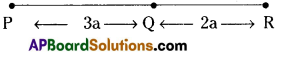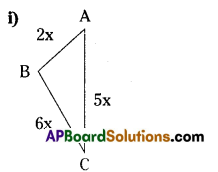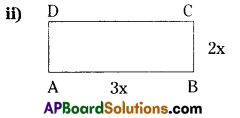# HSSlive: Plus One & Plus Two Notes & Solutions for Kerala State Board

## AP Board Class 7 Maths Chapter 10 Algebraic Expressions Ex 3 Textbook Solutions PDF: Download Andhra Pradesh Board STD 7th Maths Chapter 10 Algebraic Expressions Ex 3 Book AnswersAP Board Class 7 Maths Chapter 10 Algebraic Expressions Ex 3 Textbook Solutions PDF: Download Andhra Pradesh Board STD 7th Maths Chapter 10 Algebraic Expressions Ex 3 Book Answers

## Andhra Pradesh State Board Class 7th Maths Chapter 10 Algebraic Expressions Ex 3 Books Solutions

 Board AP Board Materials Textbook Solutions/Guide Format DOC/PDF Class 7th Subject Maths Chapters Maths Chapter 10 Algebraic Expressions Ex 3 Provider Hsslive

2. Click on the Andhra Pradesh Board Class 7th Maths Chapter 10 Algebraic Expressions Ex 3 Answers.
3. Look for your Andhra Pradesh Board STD 7th Maths Chapter 10 Algebraic Expressions Ex 3 Textbooks PDF.
4. Now download or read the Andhra Pradesh Board Class 7th Maths Chapter 10 Algebraic Expressions Ex 3 Textbook Solutions for PDF Free.

## AP Board Class 7th Maths Chapter 10 Algebraic Expressions Ex 3 Textbooks Solutions with Answer PDF Download

Find below the list of all AP Board Class 7th Maths Chapter 10 Algebraic Expressions Ex 3 Textbook Solutions for PDF’s for you to download and prepare for the upcoming exams:

Question 1.
Find the length of the line segment PR in the following figure in terms of ’a’.Solution:
The length of PR = PQ⎯⎯⎯⎯⎯⎯⎯⎯+QR⎯⎯⎯⎯⎯⎯⎯⎯
= 3a + 2a
= 5a units

Question 2.
(i) Find the perimeter of the following triangle.(ii) Find the perimeter of the following rectangle.Solution:
i) The perimeter of the triangle = AB⎯⎯⎯⎯⎯⎯⎯⎯+BC⎯⎯⎯⎯⎯⎯⎯⎯+CA⎯⎯⎯⎯⎯⎯⎯⎯
= 2x + 6x + 5x
= 13x units
ii) The perimeter of the rectangle ABCD = AB⎯⎯⎯⎯⎯⎯⎯⎯+BC⎯⎯⎯⎯⎯⎯⎯⎯+CD⎯⎯⎯⎯⎯⎯⎯⎯+DA⎯⎯⎯⎯⎯⎯⎯⎯⎯
or
= 2 (l + b)
= 2 (3x + 2x)
= 2 × 5x
= 10x units

Question 3.
Subtract the second terni from first term.
(i) 8x, 5x
(ii) 5p, 11p
(iii) 13m2, 2m2
Solution:
i) 8x – 5x = 3x
ii) 5p – 11p = -6p
iii) 13m2 – 2m2 = 11m2

Question 4.
Find the value of following monomials, if x =1.
(i) -x
(ii) 4x
(iii) -2x2
Solution:
1) If x = 1 ⇒ – x = – (1)= – 1
ii) If x = 1 ⇒ 4x = 4 x 1 = 4
iii) If x= 1 ⇒ – 2x2 = – 2(1)2 = – 2 × 1= – 2

Question 5.
Simplify and find the value of 4x + x – 2x2 + x – 1 when x = -1.
Solution:
Given expression = 4x + x – 2x2 + x – 1
= 4x + x + x – 2x2 – 1
= – 2x2 + 6x – 1
If x = – 1 then, the value of – 2x2 + 6x – 1 = – 2 (-1)2 + 6( – 1) – 1
= – 2(1) – 6 – 1
= – 2 – 6 – 1
= – 9

Question 6.
Write the expression 5x2 – 4 – 3x2 + 6x + 8 + 5x – 13 in its simplified form. Find its value when x = -2
Solution:
The given algebraic expression = 5x2 – 4 – 3x2 + 6x + 8 + 5x – 13.
= (5x2 – 3x2) (6x + 5x) + (-4 – 13)
= 2x2 + 11x – 17
When x = – 2. then the value of 2x2+ 11x – 17 = 2(- 2)2 + 11(-2) – 17
= 2 × 4 – 22 – 17
= 8 – 39
= – 31

Question 7.
If x = 1; y = 2 find the values of the following expressions
(i) 4x – 3y + 5
(ii) x2 + y2
(iii) xy + 3y – 9
Solution:
i) 4x – 3y+5
If x = 1, y = 2 then the value of 4x – 3y + 5 = 4(1) – 3(2) + 5
= 4 – 6 + 5
= 9 – 6 = 3

ii) x2 + y2
If x = 1, y = 2, the value of x2 + y2= (1)2 + (2)2
=(1 × 1) + (2 × 2)
= 5

iii) xy + 3y – 9
If x = 1; y = 2,the value of xy + 3y – 9 = 1 × 2 + 3(2) – 9
= 2 + 6 – 9
= 8 – 9

Question 8.
Area of a rectangle is given by A = l × b . If l = 9cm, b = 6cm, find its area?
Solution:
Formula for area of a rectangle (A) = l × b
If l = 9cm, b = 6cm
then the area of the rectangle = l × b
= 9 × 6
= 54 cm2

Question 9.
Simple interest is given by I = 𝑃𝑇𝑅100 . If P = ₹ 900, T =2 years; and R =5%, find the simple interest.
Solution:
Given that the formula for simple interest (I) = 𝑃𝑇𝑅100
P = Rs.900, T = 2years, R = 5%
∴ The required simple interest (I) = PTR100=900×2×5100 = 9 x 2 x 5 = Rs. 90

Question 10.
The relationship between speed (s), distance (d) and time (t) is given by S = – . Find the
value of s, if d = 135 meters and t = 10 seconds.
Solution:
Given that s = dt and d = 135 meters, t = 10 seconds
∴ The value of s = dt=13510
∴ speed (s) = 13.5 m/sec.

## Andhra Pradesh Board Class 7th Maths Chapter 10 Algebraic Expressions Ex 3 Textbooks for Exam Preparations

Andhra Pradesh Board Class 7th Maths Chapter 10 Algebraic Expressions Ex 3 Textbook Solutions can be of great help in your Andhra Pradesh Board Class 7th Maths Chapter 10 Algebraic Expressions Ex 3 exam preparation. The AP Board STD 7th Maths Chapter 10 Algebraic Expressions Ex 3 Textbooks study material, used with the English medium textbooks, can help you complete the entire Class 7th Maths Chapter 10 Algebraic Expressions Ex 3 Books State Board syllabus with maximum efficiency.

## FAQs Regarding Andhra Pradesh Board Class 7th Maths Chapter 10 Algebraic Expressions Ex 3 Textbook Solutions

#### Can we get a Andhra Pradesh State Board Book PDF for all Classes?

Yes you can get Andhra Pradesh Board Text Book PDF for all classes using the links provided in the above article.

## Important Terms

Andhra Pradesh Board Class 7th Maths Chapter 10 Algebraic Expressions Ex 3, AP Board Class 7th Maths Chapter 10 Algebraic Expressions Ex 3 Textbooks, Andhra Pradesh State Board Class 7th Maths Chapter 10 Algebraic Expressions Ex 3, Andhra Pradesh State Board Class 7th Maths Chapter 10 Algebraic Expressions Ex 3 Textbook solutions, AP Board Class 7th Maths Chapter 10 Algebraic Expressions Ex 3 Textbooks Solutions, Andhra Pradesh Board STD 7th Maths Chapter 10 Algebraic Expressions Ex 3, AP Board STD 7th Maths Chapter 10 Algebraic Expressions Ex 3 Textbooks, Andhra Pradesh State Board STD 7th Maths Chapter 10 Algebraic Expressions Ex 3, Andhra Pradesh State Board STD 7th Maths Chapter 10 Algebraic Expressions Ex 3 Textbook solutions, AP Board STD 7th Maths Chapter 10 Algebraic Expressions Ex 3 Textbooks Solutions,
Share: